# Oriented gaussoids

We enumerated all oriented gaussoids for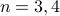:

## Enumeration details

By definition an oriented gaussoid is an assignment of symbolsto signs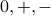which is compatible with all edge trinomials. Let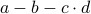be an edge trinomial with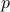-variables removed. Compatibility with this trinomial can be expressed with the formula

``` ((a=0&b=0)->(c=0|d=0))&
(((a=0&b=+)|(a=-&b=0)|(a=-&b=+))->((c=+&d=-)|(c=-&d=+)))&
(((a=0&b=-)|(a=+&b=0)|(a=+&b=-))->((c=+&d=+)|(c=-&d=-)))
```

which is a compressed listing of all compatible assignments as a case distinction on the values of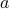and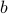. Another formula for compatibility is

```(a=+|b=-|(c=+&d=-)|(c=-&d=+))<->(a=-|b=+|(c=+&d=+)|(c=-&d=-))
```

which reads: there is a positive term if and only if there is a negative term. The latter formula is shorter and more symmetric but the former was solved faster most of the time in our tests.

To encode the three valueswe introduce two variables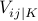and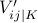for every symboland use the following translation table:0 1 1 + 1 0 - 0 1

The fourth assignment of Boolean variables is forbidden explicitly in the cnf file. Since there are twice as many variables as with gaussoids, we have to choose another ordering. If the symbolhas canonical index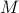, thengets index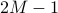andgets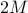.

An alternate encoding uses three variables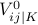,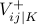, and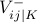for each symbol and the table:0 1 0 0 + 0 1 0 - 0 0 1

With this encoding, we can exploit the built-in support for E-clauses of the c2d solver. An E-clause is a clause in a cnf formula which must be satisfied by satisfying exactly one literal. Note that some solvers, in particular cachet, throw a parser error when they encounter an eclauses line in a DIMACS file while others, in particular sharpSAT, ignore it and compute unintended results.

The variables,, andhere get indices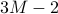,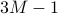and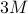respectively.

There are four possibilities to combine formula and variable encoding. The suffix “f” indicates that the second formula was used and the suffix “v” that the second variable encoding was used.

 c2d time cachet time sharpSAT time bdd_minisat_all time ocnf3 51 0.04 * 0.02 * 0.00 ocnf3-list.txt 0.00 ocnf4 34873 2.46 * 3.51 * 0.68 ocnf4-list.txt 1.62 ocnf5 OOM - 54936241913 48126 * 196298 - - ocnf3f * 0.16 * 0.02 * 0.00 - - ocnf4f * 10.42 * 3.12 * 1.97 - - ocnf5f OOM - * 129868 * 363406 - - ocnf3v * 0.06 - - - - - - ocnf4v * 1.88 - - - - - - ocnf5v OOM - - - - - - - ocnf3fv * 0.04 - - - - - - ocnf4fv * 0.84 - - - - - - ocnf5fv OOM - - - - - - -

The following perl script implements the translation table for the first variable encoding, which was used in ocnf{3,4,5}:

binary2oriented.pl
```#!/usr/bin/perl -an
my %conv = ('11' => '0', '10' => '+', '01' => '-');
chomp;
print \$conv{\$_} for m/../g;
print "\n";
```

We obtain a string composed of 0, + and - which encodes the orientation of the supporting gaussoid under the canonical numbering of-variables. The listings linked in the table were obtained with:

```\$ minisat2binary.pl <ocnf3-list | binary2oriented.pl >ocnf3-list.txt
```